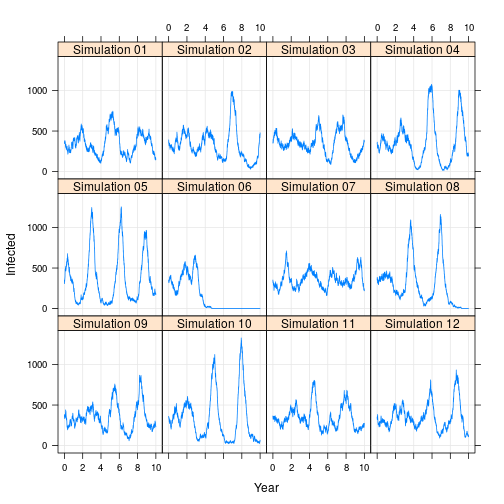## Stochastic SIR Epidemiological Compartment Model

Christian Gunning — written Apr 25, 2015 — source

### Introduction

This post is a simple introduction to Rcpp for disease ecologists, epidemiologists, or dynamical systems modelers - the sorts of folks who will benefit from a simple but fully-working example. My intent is to provide a complete, self-contained introduction to modeling with Rcpp. My hope is that this model can be easily modified to run any dynamical simulation that has dependence on the previous time step (and can therefore not be vectorized).

This post uses a classic Susceptible-Infected-Recovered (SIR) epidemiological compartment model. Compartment models are simple, commonly-used dynamical systems models. Here I demonstrate the tau-leap method, where a discrete number of individuals move probabilistically between compartments at fixed intervals in time. In this model, the wait-times within class are exponentially distributed, and the number of transitions between states in a fixed time step are Poisson distributed.

This model is parameterized for the spread of measles in a closed population, where the birth rate (nu) = death rate (mu). The transmission rate (beta) describes how frequently susceptible (S) and infected (I) individuals come into contact, and the recovery rate (gamma) describes the the average time an individual spends infected before recovering.

### C++ Code

Note: C++ Functions must be marked with the following comment for use in R: `// [[Rcpp::export]]`.

When functions are exported in this way via sourceCpp(), RNG setup is automatically handled to use R’s engine. For details on random number generation with Rcpp, see the this Rcpp Gallery post.

### R Code

Next we need to parameterize the model. Modelers often deal with many named parameters, some of which are dependent on each other. My goal here is to specify parameters in R once (and only once), and then pass all of them together to the main cpp function.

### Plot Results

Note that the model contains no seasonality. Rather, the system experiences stochastic resonance, where the “noise” of stochastic state transitions stimulates a resonant frequency of the system (here, 2-3 years). For more information see here.

Sometimes epidemics die out. In fact, for this model, they will die out with probability = 1 as time goes to infinity!tags: simulation  basics